# Recombination Dynamics in Thin-film Photovoltaic Materials via Time-resolved Microwave Conductivity

Engineering

## Your institution must subscribe to JoVE's Engineering section to access this content.

Fill out the form below to receive a free trial or learn more about access:

# Welcome!

Enter your email below to get your free 10 minute trial to JoVE!

We use/store this info to ensure you have proper access and that your account is secure. We may use this info to send you notifications about your account, your institutional access, and/or other related products. To learn more about our GDPR policies click here.

### Summary

A Time Resolved Microwave Conductivity technique for investigating direct and trap-mediated recombination dynamics and determining carrier mobilities of thin film semiconductors is presented here.

Guse, J. A., Jones, T. W., Danos, A., McCamey, D. R. Recombination Dynamics in Thin-film Photovoltaic Materials via Time-resolved Microwave Conductivity. J. Vis. Exp. (121), e55232, doi:10.3791/55232 (2017).

### Abstract

A method for investigating recombination dynamics of photo-induced charge carriers in thin film semiconductors, specifically in photovoltaic materials such as organo-lead halide perovskites is presented. The perovskite film thickness and absorption coefficient are initially characterized by profilometry and UV-VIS absorption spectroscopy. Calibration of both laser power and cavity sensitivity is described in detail. A protocol for performing Flash-photolysis Time Resolved Microwave Conductivity (TRMC) experiments, a non-contact method of determining the conductivity of a material, is presented. A process for identifying the real and imaginary components of the complex conductivity by performing TRMC as a function of microwave frequency is given. Charge carrier dynamics are determined under different excitation regimes (including both power and wavelength). Techniques for distinguishing between direct and trap-mediated decay processes are presented and discussed. Results are modelled and interpreted with reference to a general kinetic model of photoinduced charge carriers in a semiconductor. The techniques described are applicable to a wide range of optoelectronic materials, including organic and inorganic photovoltaic materials, nanoparticles, and conducting/semiconducting thin films.

### Introduction

Flash-photolysis time-resolved microwave conductivity (FP-TRMC) monitors dynamics of photo-excited charge carriers on the ns-µs timescale, making it an ideal tool for investigating charge carrier recombination processes. Understanding the decay mechanisms of photo-induced charge carriers in thin film semiconductors is of key importance in a range of applications, including photovoltaic device optimization. The induced carrier lifetimes are often functions of induced carrier density, excitation wavelength, mobility, trap density and trapping rate. This paper demonstrates the versatility of the Time Resolved Microwave Conductivity (TRMC) technique for investigating a wide range of carrier dynamic dependencies (intensity, wavelength, microwave frequency) and their interpretations.

Photogenerated charges can modify to both the real and the imaginary parts of the dielectric constant of a material, depending on their mobility and degree of confinement/localization1. The conductivity of a materialis proportional to its complex dielectric constant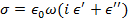whereis the frequency of a microwave electric field,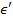and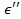are the real and imaginary parts of the dielectric constant. Thus, the real part of the conductivity is related to the imaginary part of the dielectric constant, and can be mapped onto microwave absorption, while the imaginary part of the conductivity (subsequently referred to as polarization) is related to a shift in the resonance frequency of the microwave field1.

TRMC offers several advantages over other techniques. For instance, DC photoconductivity measurements suffer from a range of complications arising from contacting the material with electrodes. Enhanced recombination at the electrode/material interface, back injection of charges through this interface, as well as enhanced dissociation of excitons and geminate pairs due to the applied voltage2 all lead to distortions in the measured carrier mobilities and lifetimes. In contrast, TRMC is an electrodeless technique which measures the intrinsic mobility of the carriers without distortions due to charge transfer across contacts.

A significant advantage of using microwave power as a probe for carrier dynamics is that, as well as monitoring the decay lifetimes of charge carriers, decay mechanisms/pathways can also be investigated.

TRMC can be used to determine the total mobility3 and lifetime4 of induced charge carriers. These parameters can subsequently be used to distinguish between direct and trap-mediated recombination mechanisms3,5. The dependence of these two separate decay pathways can be quantitatively analyzed as a function of carrier density3,5 and excitation energy/wavelength5. The localization/confinement of induced carriers can be investigated by comparing the decay of the conductivity vs polarizability5 (imaginary vs real part of dielectric constant).

Additionally, and perhaps most importantly, TRMC can be used to characterize trap states which act as charge carrier decay pathways. Surface traps, for example, can be distinguished from bulk traps by comparing passivated vs unpassivated samples6. Sub-bandgap states can be directly investigated using sub-bandgap excitation energies5. Trap densities can be deduced by fitting TRMC data7.

Due to the versatility of this technique, TRMC has been applied to study a wide range of materials including: traditional thin film semiconductors such as silicon6,8 and TiO29,10, nanoparticles11, nanotubes1, organic semiconductors12, material blends13,14, and hybrid photovoltaic materials3,4,5.

In order to obtain quantitative information using TRMC, it is crucial to be able to accurately determine the number of absorbed photons for a given optical excitation. Since methods for quantifying absorption of thin films, nanoparticles, solutions and opaque samples differ, the sample preparation and calibration techniques presented here are designed specifically for thin film samples. However, the TRMC measurement protocol presented is very general.

Subscription Required. Please recommend JoVE to your librarian.

### Protocol

1. Sample Preparation

Caution: Some chemicals used in this protocol can be hazardous to health. Please consult all relevant material safety data sheets before any sample preparation takes place. Utilize appropriate personal protective equipment (lab coats, safety glasses, gloves, etc.) and engineering controls (e.g. glovebox, fume hood, etc.) when handling the perovskite precursors, and solvents.

NOTE: The aim of this section is to form a uniform thickness thin film on the substrate. While this procedure is specific to the organo-lead halide perovskite sample, it can be modified for a range of samples and sample preparation techniques including vapor deposition, spin coating and sputtering, etc. The important outcome is a uniform thin film.

1. Cleaning the substrate
1. Place the quartz (or low iron glass) substrate in an ultrasonic bath of detergent for 30 min.
2. Repeat ultrasonic treatment with ultrapure water and then with isopropanol.
3. Place the cleaned substrates under nitrogen plasma for 30 min immediately prior to transfer into a nitrogen glovebox.
2. CH3NH3PbI3 perovskite sample preparation using the interdiffusion method15
NOTE: the following steps are performed in nitrogen glovebox.
1. Add 461 mg of PbI2 to a sample vial, and transfer to a nitrogen glovebox.
2. Add 850 µL of anhydrous dimethylformamide (DMF) to 150 µL of anhydrous dimethylsulfoxide (DMSO) to from a mixed 85:15 DMF/DMSO solvent.
3. Add the PbI2 to the DMF/DMSO solvent and heat the mixture at 100 °C while stirring with a magnetic stirrer bar until the PbI2 has completely dissolved.
4. Filter the PbI2 solution through a 0.2 µm PTFE filter into a clean sample vial and return to the 100 °C hotplate.
5. Dissolve 50 mg of CH3NH3I in 50 mL of anhydrous isopropanol.
6. Dispense 80 µL of the hot PbI2 solution onto the glass substrate (at room temperature) and immediately spin at 5,000 rpm for 30 s to form a thin PbI2 precursor film.
7. Inject a 300 µL volume of the CH3NH3I solution directly onto the center of the PbI2 film, and immediately spin coat this solution at 5,000 rpm for 30 s.
NOTE: This step should be carried out with a single confident dispense of the CH3NH3I solution. Be careful to avoid unintended drips as this affects the quality of the resulting film.
8. Place the sample on a hotplate at 100 °C for 2 h, such that the precursor film crystallizes into a perovskite structure. The resulting CH3NH3PbI3 film should be smooth, with a mirror-like surface and about 250 nm thick.
3. Sample encapsulation
NOTE: this step is only necessary for samples which suffer from atmospheric degradation.
1. Dissolve 10 mg of poly(methyl methacrylate) (PMMA) in 1 mL anhydrous chlorobenzene. Spin coat the sample with 50 µL of PMMA solution at 1,000 rpm for 30 s.

2. Sample Characterization

1. Measure sample thickness
1. Etch a small line on a companion sample. Scan the surface near this etch using a profilometer. Determine the film thickness L.
NOTE: The sample should be stored in a light free (e.g. covered in Aluminum foil) oxygen-free (e.g. nitrogen) environment until ready for use.
2. Measure the absorption spectrum
NOTE: The details of this measurement vary depending on the sample (e.g. powders vs opaque films vs semi-transparent films). The following procedure is designed for semi-transparent thin film samples. The aim of this section is to determine the wavelengths of interest to investigate (e.g. determine band-gap, excitonic features, etc.), and to calculate Fa, the fraction of absorbed photons vs incident photons at each wavelength of interest.
1. Place the sample substrate (e.g. glass slide) in the sample holder of an appropriate spectrophotometer. Record background reflectance (R(λ)) and transmittance (T(λ)) spectra as per the manufacturer's instructions. Note: a reflectance standard such as BaO4 may also be used to obtain an accurate baseline.
2. Replace the substrate with the sample and record the reflectance (R(λ)) and transmittance (T(λ)) as per the manufacturer's instructions. Subtract the background measurement to obtain accurate spectra.
NOTE: For opaque samples, a spectrophotometer with an integrating sphere attachment must be used. The diffuse reflectance is measured as in section 2.2.1-2, however the sample must be placed in at the back of the integrating sphere, as per manufacturer's instructions.
3. Calculate the absorption coefficient via: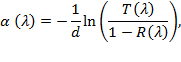NOTE: Where, d is the thickness of the film in cm.
4. Calculate the number of absorbed vs incident photons viaNOTE: Make sure the absorption coefficient and sample thickness L have the same units.
5. Determine wavelengths of interest from the absorption spectrum by inspection. These may include optical transitions or wavelengths at the band edge or in the band tail. Note the Fa at each of these wavelengths.
NOTE: The following calibration processes should be performed just prior to the experiment.

3. Laser Power Calibration

NOTE: In this section, refer to the optical excitation schematic in Figure 3. Tunable wavelength lasers like OPOs require coupling at each wavelength.

1. Couple the free-space laser into a fiber
NOTE: If the available laser is already fiber coupled, skip this section.
NOTE: Off-axis parabolic mirror fiber couplers are achromatic, which means that all wavelengths incident on the mirror are focused to the sample point. As a result, the fiber can be coupled to the free space laser at one wavelength, and does not require adjustments at each wavelength. This step should be done before any other measurements are performed
NOTE: It is possible to design a TRMC cavity and optical setup using a free space laser, although accurately and reproducibly characterizing the absorbed laser power may be slightly more difficult.
1. Set the incident wavelength to the desired value (e.g. 750 nm) as per the manufacturer's protocol. For fixed wavelength lasers, this step is unnecessary.
2. Check the laser beam profile for visible cross-beams. If these exist, use irises to allow only the central Gaussian beam to pass to the fiber coupler.
3. Align an off-axis parabolic mirror fiber coupler so that the incident laser beam is aligned with the optical axis of the mirror.
4. Connect the optical fiber to the fiber coupler and to a power sensor. The larger the fiber core, the more light can be coupled into the fiber. A 1 mm core NA 0.48 fiber works effectively.
5. Maximize fiber coupling at low power by monitoring the output power of the fiber with the power sensor while adjusting the tilt and angle of the fiber coupler. Optimum coupling is achieved when the power measured by the sensor is maximized (i.e. any adjustments of the fiber coupler tilt angle result in a lower power measurement)
NOTE: If the alignment is poor, it is possible to damage the outer cladding of the fiber. A ticking sound indicates that a hole is being burned in the cladding. In this case, immediately turn off the laser and perform a coarse alignment of the coupler at low power.
6. Gradually increase the laser power and refine the coupling as in 3.1.5.
2. Measure cavity loss factor
NOTE: This section should be performed after the fiber coupling procedure outlined in section 3.1.
1. Measure the power transmitted through the fiber using an appropriate power sensor. This measurement is performed before connecting the fiber into the cavity.
2. Measure the power at the sample. This is easiest if the cavity is made up of 4 quarter wave plates screwed together (see Figure 4). To do this accurately and reproducibly, unscrew the cavity, place a mask the size of the sample holder at the sample position and measure the laser power reaching the detector through the mask.
3. Calculate the cavity loss factor by dividing the laser power measured at the fiber by the power measured at the sample. This measurement takes into account geometrical losses as well as losses due to diffusive components in the setup.
4. Repeat this measurement for each wavelength of interest.

4. Mounting the Sample into the Cavity

1. Place the sample in a Teflon sample holder, designed such that the sample is centered in the cavity once inserted.
2. Insert the sample holder into the cavity at a location of maximum electric field, with the thin film facing the optical input of the cavity. Figure 4 shows a detailed schematic of the cavity and sample holder.

5. Cavity Sensitivity Calibration14

NOTE: Excess photo generated charge carriers lead to a change in sample conductivity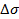(Sm-1) which results in a decrease in the microwave power reflected from the cavity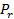. For small changes in conductivity17, the change in microwave power is proportional to the change in conductivity via a cavity sensitivity factor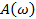: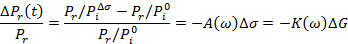The change in conductivity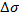of the sample is related to the change in the bulk conductancevia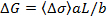NOTE: This calibration is necessary for converting microwave power to charge carrier mobility. If the aim of the study is to compare dynamics or obtain relative results, this calibration is not required.
NOTE: In this section, refer to the microwave detection setup in Figure 5.

1. Connect port 1 of a network analyzer to the circulator input port 1. Connect port 2 of a network analyzer to the point in the circuit just before detection (e.g. to the output of a detection diode or IQ modulation detector). Measure the power reflected from the loaded cavity (i.e. with the sample inserted) as a 2-port S21 measurement, in order to obtain the resonance curve of the circuit.14
NOTE: If the cavity has not been matched with the external microwave detection circuitry, the resonance curve will be different for the stand-alone cavity vs the cavity in the circuit. Thus, it is better to measure the resonance not as a one port reflection measurement from the cavity, but rather as a 2-port 'reflection' measurement through the circulator.
NOTE: The resonance frequency is mainly determined by the geometry of the cavity being used. Typical resonance frequencies for TRMC are found in X-band (~10 GHz) and Q-band (~34 GHz), although any microwave frequency can in principle be used. In this manuscript, we use a cavity with a resonance frequency of ~ 6.5 GHz, which provides a similar microwave response whilst providing a larger sample space when compared with an X-band cavity.
2. Optimize the quality factor,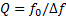, of the cavity with the tuning screw by observing the resonance dip become deeper and narrower.
NOTE: Optimizing the Q factor does not necessarily mean maximizing the Q. While increasing the Q factor increases sensitivity, the cavity response time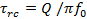also increases. It may be preferable to reduce sensitivity to obtain higher temporal resolution. If the photo-induced charge carriers significantly modify the dielectric constant of the material, the resonance frequency may also temporally shift outside of the cavity bandwidth if the Q is large, resulting in a distorted power measurement. In these cases, overcoupling the resonator slightly may improve accuracy of the reflected power.
3. Measure and record the optimized resonance curve of the using a network analyzer as described in section 5.1.1.
4. Plot Preflected/Pincident on a linear scale and fit the baseline corrected figure with a Lorentzian lineshape, as shown in Figure 6.
5. Calculate the loaded quality factor, via: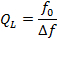NOTE: Where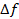is the full width at half maximum (FWHM) of the resonance curve and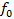is the resonance frequency.
6. Calculate the cavity sensitivity factor A (Ω cm) of the cavity via14: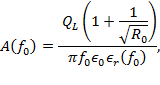where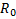is the ratio of reflected to incident power at the resonance frequency,is the loaded resonance frequency,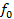is the resonance frequency,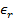is the dielectric constant of the material at the resonance frequency,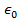is the permittivity of free space (F/cm).
NOTE: This formula assumes the sample fills the whole cavity.
7. Correct the sensitivity factor for sample geometry:
The following correction factors apply for a thin film sample of size [w × w × L], (L<<d), centered in the cavity at z0=d/4 (i.e. at the maximum electric field). Here, L is the sample thickness (cm), a and b are the long and short sides of the rectangular cavity respectively and d is the length of the cavity (cm). The geometry corrected sensitivity factor Ã is given by: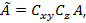where Cz, Cxy are the correction factors due to incomplete filling of the cavity space along the z and xy direction, given by: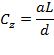6. Single TRMC Transient Measurement Procedure

1. Determine optimal measurement parameters: manually find signal
NOTE: Please refer to the experimental schematic presented in Figure 2 before reading the following sections of the protocol.
NOTE: setting up the microwave detection circuitry can be done either by hand or using the appropriate software. Typically, for each new sample, the measurement parameters (such as resonance frequency, microwave power, trigger position and time-base) are unknown and must be adjusted to identify/optimize the signal. This is usually done manually. Once the signal has been identified, the measurement parameters are then entered into a MATLAB (or other) script used to automate the measurement process.
1. Tune the laser to a wavelength of interest, as determined in section 2.2.5.
2. If the laser has an adjustable power setting, set the output power to maximum, as per the manufacturer's instructions. (This may involve manually adjusting a power knob, or may be done via software depending on the laser).
3. Connect the (already coupled) optical fiber to a power sensor, and measure the laser power transmitted through the fiber using a power meter. The fiber is not connected to the cavity at this stage.
NOTE: For very short pulsed lasers, this is often best done using thermal (average power) sensors rather than diode sensors, which may undergo temporal saturation or even dielectric breakdown at very high powers.
4. Use neutral density (ND) filters to attenuate the laser power to a desired power level.
NOTE: it is possible to set the power to a lower level and not use filters, but a more accurate power reading can be obtained by measuring a high power then attenuating.
5. Calculate Nph, the number of absorbed photons/cm2/pulse at this excitation intensity via: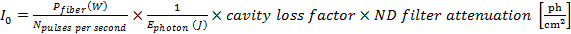6. Connect the fiber to the cavity.
7. Set up the detection circuitry as shown in Figure 5.
NOTE: A vector network analyzer was used to perform these measurements; however it is possible to use an alternative microwave detection setup, for example using a microwave diode as a power sensor.
8. Set the microwave source frequency to the resonance frequency of the loaded cavity, as measured in section 5. For our setup using the network analyzer, this involves enabling the continuous frequency output and manually entering the frequency of the output microwaves.
9. Set the microwave power to 0 dBm.
10. Trigger the network analyzer (or alternative detector) using the laser. Determine the trigger offset necessary to capture the rise of the signal with a few microseconds of 'dark' signal before the laser pulse to use as a baseline for fitting. Setting the trigger offset to 1/10 of the signal length works well (e.g. if the signal is 100 µs long, then the baseline trigger should be offset by 10 µs). This involves changing the trigger mode to "external", and adjusting the trigger offset until the signal is found.
11. Adjust the timebase of the network analyzer (or alternative detector) such that the transient tail is much longer that the initial decay. Often, there is a long tail which persists even when it appears (on a linear scale) that the signal has decayed to the noise floor.
NOTE: To determine if the timebase used is sufficiently long, record an averaged TRMC transient and then plot on a log-log scale.
2. Measure raw transient
NOTE: Typically, when obtaining suites of TRMC data, the measurement process is automated by interfacing with the microwave source and detector. In this paper, a home-made MATLAB script has been used to set the microwave output (freq, and power) and also to configure the measurement acquisition (measurement time base, trigger offset, number of averages).
1. If the measurements are automated, input the microwave frequency and power as well as the acquisition trigger offset and measurement time base which have been determined in the section above into the experiment script.
2. While continuously pulsing the laser, measure and record a TRMC decay transient on a network analyzer (or alternative detector). Average at least 100 traces (even if the S/N is very high with a single shot measurement) to compensate for shot-to-shot power variations in the pulsed laser. If the measurements are automated, this is done by executing the experiment script.
NOTE: Averaging may be required to obtain sufficient signal-to-noise, especially for samples with long, small amplitude decay tails such as shown in Figure 7.
NOTE: Inverted transients, or transients with positive and negative 'lobes', may indicate that the microwave frequency is not at the cavity resonance frequency. Adjust the source frequency until the transient signal is maximized.
3. Disconnect the fiber from the cavity and cap the optical port. Take a background reading with the same number of averages as in the previous step, with the sample still in the resonator, but no longer being illuminated.
4. Subtract the background trace from the signal trace.
3. Process raw data into mobility per charge carrier
1. Calculate change in reflected power via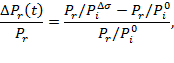NOTE: Where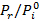is the baseline value of the raw transient (before illumination) and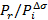is the raw transient data.
NOTE: If the detector measures voltage not power (e.g. Diode + oscilloscope), then a scaling factor must be included. The scaling factor is usually quoted by the diode manufacturer; otherwise it may be obtained by performing a calibration of output voltage vs input microwave power.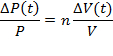2. Convert the change in reflected power to mobility per charge carrier (i.e. rescale transient) via: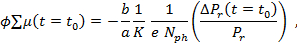NOTE: Where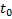corresponds to the end of the laser pulse,is the charge of an electron,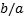is the ratio between the short and long dimensions of the cavity and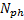is number of absorbed photons per cm2 and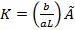(Ω) related the reflected microwave power to the change in conductance ΔG. This rescaling allows for the meaningful comparison of TRMC transients taken at different laser powers and wavelengths.
NOTE: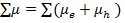is actually the total mobility of electron and holes. However, we cannot distinguish between these contributions using TRMC, and therefore we lump them together for simplicity.
3. Fit the TRMC trace with an appropriate model.
NOTE: This is simple if the data follows a single or double exponential form. However, it the data does have a simple form it may be necessary to fit the data to a kinetic model, which involved fitting the solution of an ODE (see Figure 7). The fitting equation/model should be convolved with an instrument response function (e.g. Gaussian centered at t=tlaser with a width corresponding to the response time of the instrument which limits the temporal resolution of the data.)

7. Investigating the Real and Imaginary Components of Conductivity

1. Measuring TRMC traces as a function of microwave probe frequency
NOTE: The (complex) conductivity dynamics can be deconstructed into the real (conductivity) and imaginary (polarization) components by taking multiple TRMC traces at microwave frequencies spanning the resonance curve of the loaded cavity.
1. Determine the resonance frequency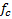of the cavity with the sample in the dark from the S21 cavity resonance curve (see Figure 6).
2. Choose x>20 frequency pointsalong this resonance curve. These points will be used to fit a Lorentzian function, so it is best if there are more points close to the dark resonance frequency fc (see Figure 9).
3. Set the excitation wavelength depending on the polarization dynamics of interest (e.g. above bandgap for free carrier polarization, sub-bandgap for trapped charge polarization).
4. Set the laser power to maximum (this will give the highest S/N).
5. Measure the laser power out of the fiber. Set the probe microwave frequency to the resonance frequency of the cavity in the dark.
6. Obtain a TRMC trace as described in section 6. Repeat the measurement described above at a fixed laser intensity for.
2. Frequency data post-processing: deconstruction into real and imaginary parts
1. Plot TRMC transient power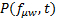as a function time and probe microwave frequency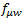, as shown in Figure 8.
2. Plot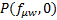and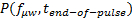, the TRMC power at t=0 and at t=end of laser pulse for each microwave frequency, as shown in Figure 8.
3. For each slice in time, construct a resonance curve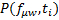.
4. Fit this curve with a Lorentzian to obtain the resonance frequency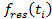, and the resonant power.
5. Plotvsto obtain a hysteresis-like polarization evolution plot (see inset in Figure 8).
6. Calculate the normalized transient frequency and transient power shift via: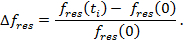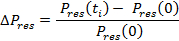7. Plot the change in resonance frequency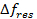, change in resonance power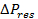and change in transient power at the cavity center frequency, as shown in Figure 10.

8. Intensity Dependent Data Suite

1. Tune the laser to a wavelength of interest, as determined in section 2.2.5.
2. Set the laser power to maximum.
3. Measure the laser power out of the fiber.
4. Connect the fiber to the cavity.
5. Obtain a single TRMC transient as outlined in section 6.
6. Insert an ND filter anywhere between the laser and the fiber (either between two irises, or just before the fiber coupler. For lasers with a fiber output, the ND filter must be placed between the fiber output and the cavity optical port).
7. Calculate and record the modified number of absorbed photons as described in 6.1.5.
8. Obtain a single TRMC transient as outlined in section 6.
NOTE: as the attenuation increases, it will become necessary to increase the number of averages.
9. Repeat 8.6-8.8 for as many combinations of ND filters as required.
NOTE: Intensity dependencies are often observed over several orders of magnitude. The high power limit is set by the maximum output laser power at a given wavelength. The low power limit is set by the sensitivity of the detection setup.

9. Wavelength Dependent Data Suite

NOTE: In order to compare TRMC transients at different wavelengths, the laser must be calibrated at each wavelength such that then induced carrier concentration is constant.

1. Determine the wavelength which limits the maximum achievable induced charge carrier density Ncarriers. This may be limited by the laser power available at that wavelength or by the absorption properties of the sample. For example, when measuring TRMC transients at wavelength spanning the above, inter and sub-bandgap regime, the low absorption at sub-bandgap wavelengths will limit the maximum carrier density.
2. Calculate the laser power necessary to generate this constant reference carrier density Ncarriers at each wavelength using: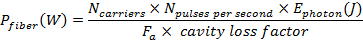3. Tune the laser to the desired wavelength. Set the laser power to the value calculated in 9.2. Connect the fiber to the cavity. Obtain a single TRMC transient as outlined in section 6. Repeat step 9.3 for each wavelength of interest.

Subscription Required. Please recommend JoVE to your librarian.

### Representative Results

The representative results presented here were obtained from a 250 nm CH3NH3PbI3 thin film sample.

The dynamics of the conductivitycan be related to the dynamics of the charge carriers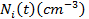viaassuming that the charge carrier mobilities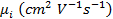are constant in time, at least on the timescale of the decay. Furthermore, assuming no recombination of the initially formed charge carriers occurs during the laser pulse, the maximum (end-of-pulse) change in conductance can be written as:whereis the charge of an electron,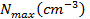is the maximum photoinduced charge carrier density,is the photon intensity incident on the sample (photons/cm2),is the internal quantum efficiency and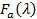is the fraction of incident light absorbed at a given wavelength, which can be calculated from the absorption spectrum (see Figure 1). The TRMC peaktherefore corresponds to the intrinsic (microwave) charge carrier mobility of the sample.

It is important to note that the quoted conductivity is an average conductivity throughout the film14,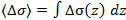. The description above assumes a homogenous photoinduced carrier density throughout the sample, which is valid for samples with a low enough optical density so that power dissipation is generally uniform throughout the sample. While induced charge carrier inhomogeneity (which can be approximated via the Beer-Lambert law) complicates the analysis of the photoinduced conductivity gradient, it does not does not affect the correct quantization of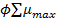, since the total change in conductance is independent of carrier concentration gradient. However, non-uniform carrier concentration may affect higher order nonlinear processes in the sample.

A general model with direct and one-level trap-mediated recombination is shown below7.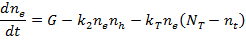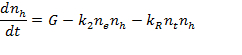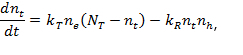whereare the electron hole and trap populations,is the generation rate,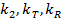are the bimolecular recombination rate, the trapping rate and the trap recombination rate respectively. By fitting TRMC data with a kinetic model such as the one described above convoluted with a Gaussian instrument response function, it is possible to not only determine carrier lifetimes and trap densities, but also characterize direct and trap-mediated recombination processes. Figure 7 shows a representative fit using the parameters in Table 1.

Special care should be taken in determining the uniqueness of fit parameters. It is useful if complimentary experiments can be performed to validate one of the timescales (e.g. time resolved PL measurement can be used to obtain the direct recombination rate.

If a decay tail is present in the data, it is important to obtain data for long enough to accurately represent this tail decay: fitting the same data cropped to a shorter timescale may result in different timescales. Low intensity decay tails can be especially difficult to fit accurately if the tail disappears into the noise floor of the instrument.

For our setup, the temporal resolution is limited by the response time of the vector network analyzer, which has a response time of about 60 ns. The Q factor for the measurements presented here is about 150, with a corresponding cavity response time of approx. 7 ns. For alternative microwave detection setups with a faster response time (e.g. microwave diode and oscilloscope), the cavity lifetime may limit the temporal resolution.

The microwave frequency dependence of TRMC decay can be used to deconstruct complex conductivity into its real (conductivity) and imaginary (polarizability) components. Figure 8 shows the raw TRMC traces taken as a function of probe microwave frequency, spanning 23 frequencies across the resonance curve of the dark cavity. On the left are 3 representative traces (raw data). Note that data taken off resonance may display a distorted decay profile, with positive or negative lobes, or even become inverted. On the right is a 3D representation of microwave power as a function of time and frequency P(t, f). At t=0, the TRMC baselines reconstruct the resonance curve of the loaded cavity. A maximal shift in both the power and frequency of the reflected microwaves occurs at t ≈ 7 µs (end-of-pulse).

The dynamic shift in the resonance curve of the cavity as a result of excess photoinduced charge carriers is shown in Figure 9. This shift may or may not be significant, depending on the dielectric properties of the material (i.e. if the complex conductivity has an imaginary component or not). The red trace is reconstructed from the baseline of TRMC traces taken at multiple microwave probe frequencies. This trace corresponds to the dark cavity resonance. The blue trace is reconstructed from the end-of-pulse TRMC power taken at multiple frequencies. The inset shows the excursion of the resonance frequency vs resonant power during the decay.

Polarization dynamics can be used to distinguish between direct and trap-mediated recombination pathways. Figure 10 shows a deconstruction of a TRMC trace into contributions from the real and imaginary components of the conductivity. The red trace is TRMC data taken at a fixed frequency, the resonance frequency of the dark loaded cavity. This is a typical TRMC measurement of complex conductivity. The decay of the real part of the conductivity (resonant power obtained from fitting) is plotted in the green trace. The decay of the polarization (the resonance frequency) is shown in blue. The polarization decay exhibits a significantly smaller decay tail than the conductivity decay. This implies that at long times, the charge carriers are contributing more to conductivity then they are to polarization, which is consistent with decay via localized trapped states.

Second order interactions of photo-induced charge carriers can be investigated via the excitation intensity dependence of charge carrier dynamics. Figure 11a shows TRMC traces taken at 530 nm excitation at various excitation intensities spanning two orders of magnitude, from 1012 - 1014 absorbed photons/cm2. The TRMC traces exhibit two distinct timescales, indicating the presence of two distinct decay mechanisms: The fast decay (which occurs on the order of 100 ns) is attributed to direct recombination processes while the long tail decay is attributed to trap-mediated recombination. While the signal typically increases with increasing laser power, the mobility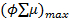of the CH3NH3PbI3 perovskite decreases with increasing excitation intensity, as shown in Figure 11b. The intensity (and therefore carrier density) dependence is sub-linear, indicating the presence of higher order decay processes.

The dependence of charge carrier decay pathways on incident photon energy (wavelength) is shown in Figure 12. Wavelengths of 530, 750 and 780 nm were chosen to represent the above, inter and sub-bandgap regime, as determined from the absorption spectrum (Figure 1). Taking into account the different absorption coefficients at these wavelengths, the TRMC traces were taken at laser powers which resulted in an absorbed photon density of 5 × 1012 photons/cm2 for each trace. It is clear that while the decay timescales are independent of excitation wavelength, the number of accessible trap states (which contribute to the decay pathway responsible for the long decay tail) is larger for above band-gap states then for those close to the bandgap.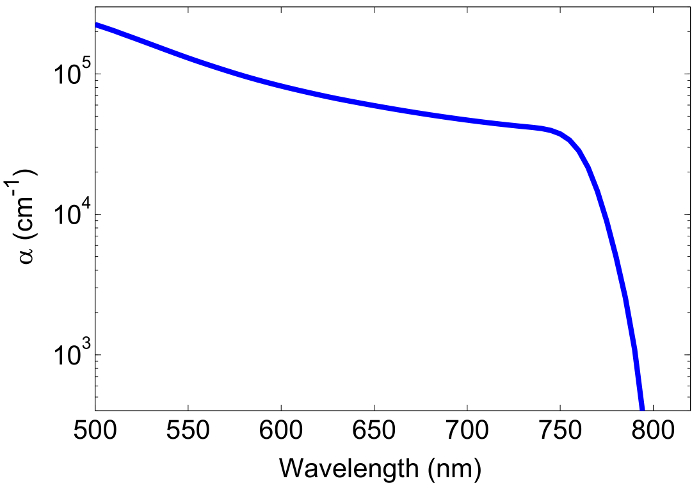Figure 1: Absorption spectrum of CH3NH3PbI3. The absorption spectrum is used to both determine the spectral area of interest, as well as for calibration of the absorbed laser power. The bandgap of this sample is about 750 nm, with tail states extending to 780 nm. Wavelengths of interest may include: above bandgap regime (λ<700 nm), bandgap (λ=750 nm) and tail state region (750 nm<λ<780 nm). Please click here to view a larger version of this figure.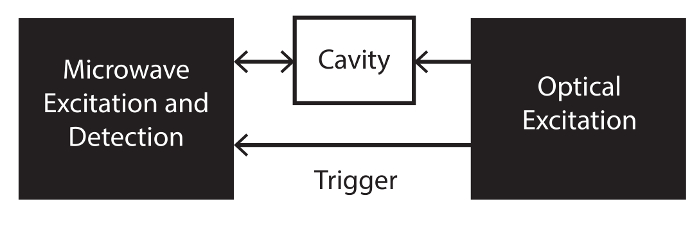Figure 2: General TRMC experimental schematic. A sample placed in a microwave cavity is photoexcited via the optical excitation setup, while being probed with microwave detection circuitry. The laser provides a trigger for measurement synchronization. Please click here to view a larger version of this figure.Figure 3: Optical setup. A tunable wavelength laser is used to optically excite charge carriers in a sample placed in a microwave cavity. A parabolic mirror is used to couple the free space laser into an optical fiber. Neutral density filters are used to obtain well calibrated laser power series. Two irises are used to eliminate cross-beams which can damage the fiber cladding. Please click here to view a larger version of this figure.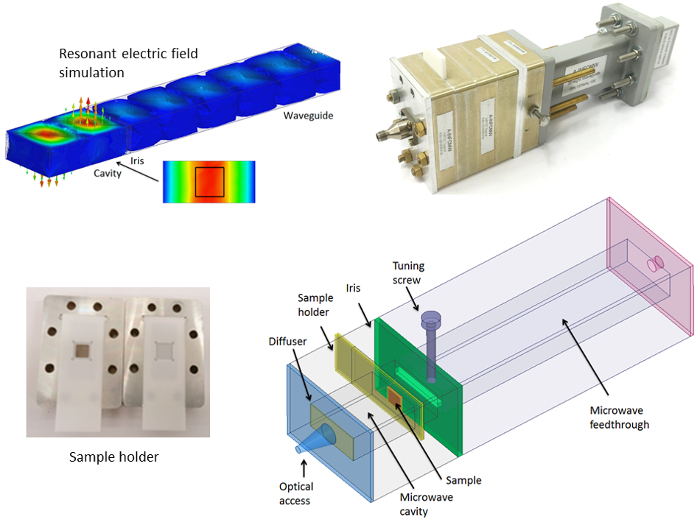Figure 4: Microwave cavity. Top right: photo of the cavity. Top left: HFSS cavity simulation shows that the electric field is approximately uniform and maximum at the sample position. Bottom: model of the cavity. A small hole in the microwave short allows optical access into the cavity. A Teflon diffuser is used to ensure the light incident on the sample is spatially uniform. The sample is placed in a Teflon sample holder at the maximum electric field position. An iris is used to define the front end of the cavity. A tuning screw is used to optimize the Q factor of the cavity. Please click here to view a larger version of this figure.Figure 5: Microwave detection schematic. A vector network analyzer is used as both a microwave source and as an IQ detector. The output microwave power is split into two paths: an excitation arm and a detection arm. The microwave excitation passes through a circulator into a cavity, where it interacts with a sample. Reflected microwave power passes through the circulator into an amplifier before entering the detector. The signal is split in two, and half is mixed with the original microwave signal (yielding the in-phase signal I) and the other half is mixed with the original microwave signal phase shifted by 90° (yielding the quadrature component of the signal Q). Finally, the amplitude of the signal is calculated via.

The vector network analyzer can be used both in time domain (to obtain TRMC traces at a fixed frequency) and in frequency domain (to obtain a steady state S21 cavity reflection curve), without changing the loading on the cavity. Please click here to view a larger version of this figure.Figure 6: Resonance curve of loaded cavity. A linear scale resonance curve used to calculate the cavity sensitivity factor. The Lorentzian fit (blue) is used to extract the resonance frequency, FWHM bandwidth and minimum reflected power R0. Please click here to view a larger version of this figure.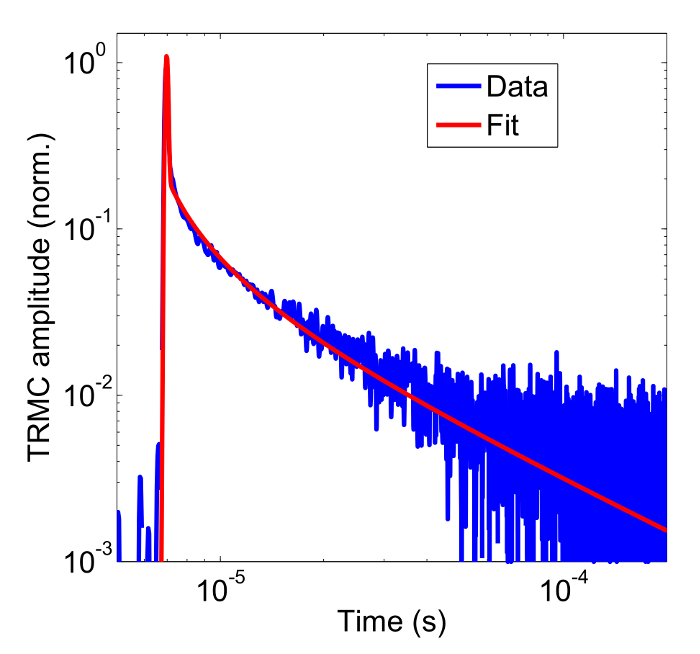Figure 7: Fit with ODE. Normalized TRMC trace, fit with a kinetic model convoluted with a Gaussian instrument response function describing direct and trap-mediated recombination processes. The data is shown on a log-log plot to highlight the existence of a low amplitude tail decay. The laser pulse occurs at t ≈ 7 × 10-6 s. Please click here to view a larger version of this figure.

 fit parameter k2 (cm3 s-1) kT (cm3 s-1) kR (cm3 s-1) NT (cm3) value 6.50×10-10 7.90×10-8 1.10×10-9 1.60×1016

Table 1: Fitting parameters. Fit parameters of TRMC trace taken at 530 nm excitation with 6.4 x 1014 absorbed ph/cm2.Figure 8: Microwave frequency series. Raw TRMC traces taken as a function of probe microwave frequency, spanning 23 frequencies across the resonance curve of the dark cavity. On the left are 3 representative traces (raw data). On the right is a 3D representation of microwave power as a function of time and frequency P(t, f). A t=0, the TRMC baselines reconstruct the resonance curve of the loaded cavity. At t ≈ 7 µs (end-of-pulse), there is a pronounced shift in the resonance curve. Please click here to view a larger version of this figure.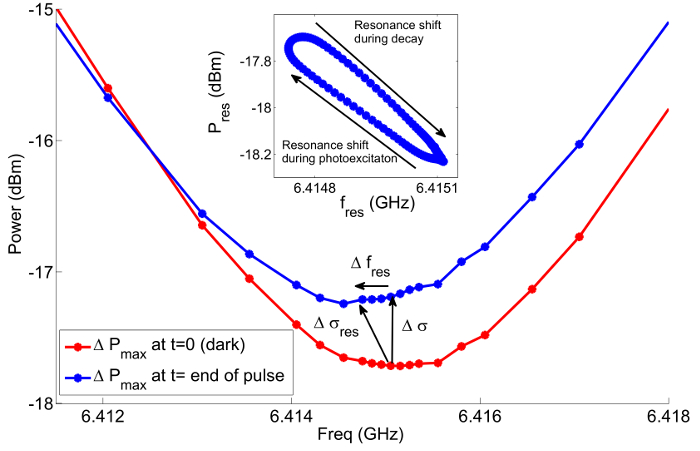Figure 9: Photoinduced cavity resonance shift. Resonance curves reconstructed from TRMC traces before (red) and just after (blue) illumination. The resonance curve shifts both in amplitude (change in real conductivity) and in frequency (change in imaginary conductivity). The inset tracks the evolution of the resonant power vs frequency during the decay. Please click here to view a larger version of this figure.Figure 10: Decay dynamics of real vs imaginary conductivity. The red trace is TRMC data taken at a fixed frequency fc, the resonance frequency of the dark loaded cavity. The green trace is the change in power at the resonance frequency, obtained from fitting. The blue trace is the change in resonance frequency as a function of time. Please click here to view a larger version of this figure.Figure 11: Intensity series. Left: The intensity dependence of sample mobilitytaken at various excitation wavelengths between 530 and 780 nm. Right: TRMC transients taken as a function of laser power. ND filters are used to attenuate the laser power by a known amount. The intensity corresponds to the number of absorbed photons/cm2. The laser pulse occurs at t ≈ 7 × 10-6 s. Please click here to view a larger version of this figure.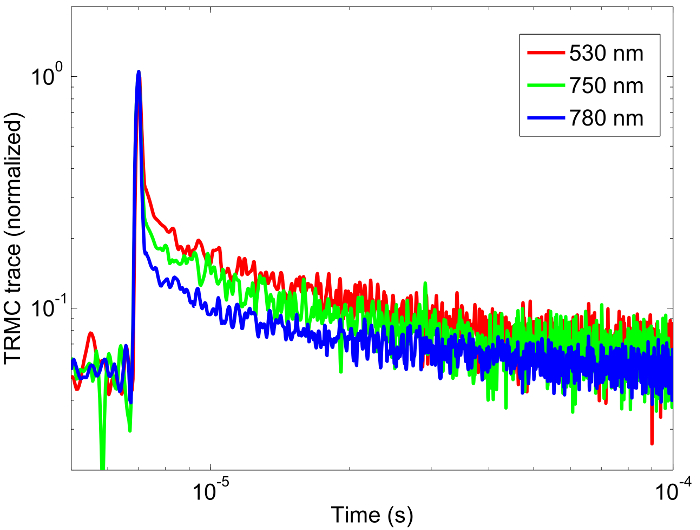Figure 12: Wavelength series. Normalized TRMC traces taken at a fixed photon density of 5 × 1012 absorbed photons/cm2 at excitation wavelength of 530, 750 and 780 nm. The laser pulse occurs at t ≈ 7 × 10-6 s. Please click here to view a larger version of this figure.

Subscription Required. Please recommend JoVE to your librarian.

### Discussion

While the TRMC technique can offer a wealth of information about photoinduced charge carrier dynamics, this is an indirect measurement of conductivity, and therefore care needs to be taken when interpreting results. The TRMC technique measures total mobility, and cannot be used to distinguish between electron and hole mobilities. The underlying assumption that conductivity is proportional to change in reflected power holds only when that change is small (< 5%)16. Furthermore, if the shift in resonance frequency during the decay is large, then the total (complex) conductivity will have to be deconstructed into its real and imaginary components before the data can be analyzed. The TRMC technique is sensitive to changes in the imaginary part of the dielectric constant, which may have contributions not only from electrical conductivity but also from dielectric loss due to dipole reorientation. This technique cannot be used to distinguish between these two mechanisms, and we assume here that electrical conductivity is the dominant contribution to the imaginary dielectric constant, which is a good assumption for crystalline materials, but may not be valid for samples in solution.

In order to obtain absolute rather than relative measurements, the TRMC technique requires extensive calibration. In particular, calibrating the optical setup to determine the absorbed photons/incident photons is crucial to obtain accurate mobilities. In principle, it is possible to use quantitative TRMC on liquid or powdered samples; however accurate characterization of the absorption of these samples can be difficult.

The calibration of the cavity sensitivity factor may also be difficult if the dielectric constantof the material is unknown. In this case, the cavity sensitivity must be obtained either by modelling the reflection parameters of the cavity using a high frequency electromagnetic simulator1,14, or using a thin (<1 μm) calibration sample which has a similar loading on the cavity as the sample under test. If the cavity sensitivity cannot be measured, it is possible to obtain meaningful relative measurements (e.g. as a function of intensity or wavelength) and extract dynamic information.

AC mobility measurements may be several orders of magnitude higher than those obtained by DC measurements much as time of flight (TOF) or photo-CELIV measurements. For example, the DC mobility of polymer matrices is dominated by inter-chain transport, leading to a mobility orders of magnitude smaller than that obtained using TRMC17. This is because DC measurements yield an effective mobility through a device, while AC mobilities are intrinsic mobilities of the material, unaffected by material-contact interactions, or perturbations of the thermal drift velocity of charge carriers due to large driving voltages. DC and AC mobility measurements may be used in tandem to investigate charge transport through photovoltaic or electro-luminescent devices: TRMC measurements elucidate intrinsic charge carrier transport mechanisms, while DC measurements can be used to identify the dominant transport mechanism of the material in a device.

A very useful extension to the TRMC experiment is the addition of a time resolved photoluminescence setup to monitor the decay of charge carriers via direct recombination. In this way, the PL measurement can be used to unambiguously distinguish the direct recombination pathway from other decay mechanisms which contribute the TRMC decay, and significantly speed up the fitting procedure.

There are several extensions to the TRMC technique. For instance, Field induced TRMC, in which TRMC measurements are performed on a device under an electric field bias which provide a steady state injection of carriers, may be used to probe interfacial trap sites in the device18.

Some of the limitations of the TRMC technique can be overcome by comparing several samples.

For instance while a single TRMC measurement cannot distinguish between electron and hole mobilities, it is possible to compare a neat sample with a sample placed in an electron or hole accepting layer3. Additionally, TRMC cannot be used to distinguish between surface or bulk traps, however it is possible to compare unpassivated vs passivated samples to determine the contribution of surface traps to the trap-mediated decay process6. Alternatively, a series of thin films with increasing thickness could be used to determine if there is a surface/volume ratio dependence on the trap density.

Subscription Required. Please recommend JoVE to your librarian.

### Disclosures

The authors have nothing to disclose.

### Acknowledgments

Acknowledgment is made to the Australian Research Council (LE130100146, DP160103008). JAG is supported via an Australian Postgraduate Award, and DRM by an ARC Future Fellowship (FT130100214). We thank Nikos Kopidakis for helpful discussions.

### Materials

 Name Company Catalog Number Comments Hellmanex III detergent Sigma Aldrich www.sigmaaldrich.com/catalog/product/sial/z805939?lang=en®ion=AU Z805939 Corrosive and toxic. See SDS. Lead(II) iodide (99%) Sigma Aldrich www.sigmaaldrich.com/catalog/product/aldrich/211168?lang=en®ion=AU 211168 Toxic. See SDS. Anhydrous dimethylformamide (99.8%) Sigma Aldrich www.sigmaaldrich.com/catalog/product/sial/227056?lang=en®ion=AU 227056 Toxic. See SDS. Anhydrous dimethylsulfoxide (99.9%) Sigma Aldrich www.sigmaaldrich.com/catalog/product/sial/276855?lang=en®ion=AU 276855 Toxic. See SDS. Anhydrous 2-Propanol (99.5%) Sigma Aldrich www.sigmaaldrich.com/catalog/product/sial/278475?lang=en®ion=AU&gclid= COnlgPaw780CFQZvvAod17EA4Q 278475 Methylammonium iodide Dyesol www.dyesol.com/products/dsc-materials/perovskite-precursors/methylammonium-iodide.html MS101000 Also sold by Sigma Aldrich Poly(methyl methacrylate) Sigma Aldrich 445746 Anhydrous chlorobenzene (99.8%) Sigma Aldrich www.sigmaaldrich.com/catalog/product/aldrich/445746?lang=en®ion=AU 284513 Toxic. See SDS. Equipment Company Model Comments/Description UV-VIS-NIR spectrophotometer Perkin-Elmer Lambda 900 Profilometer Veeco Dektak 150 Vector Network Analyzer Keysight www.keysight.com/en/pdx-x201927-pn-N9918A/fieldfox-handheld-microwave-analyzer-265-ghz?cc=US&lc=eng Fieldfox N9918A Tunable wavelength laser Opotek www.opotek.com/product/opolette-355 Opolette 355 Neutral density filters Thorlabs www.thorlabs.hk/newgrouppage9.cfm?objectgroup_id=3193 NUK01 Power meter Thorlabs www.thorlabs.com/thorproduct.cfm?partnumber=PM100D PM100D Power sensor Thorlabs www.thorlabs.com/thorproduct.cfm?partnumber=S401C S401C Cavity Custom built The cavity used in for this experiment was designed and built in-house.